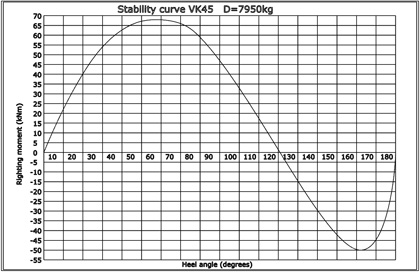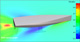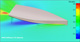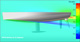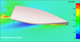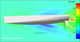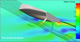Watch dynamic videos

Sails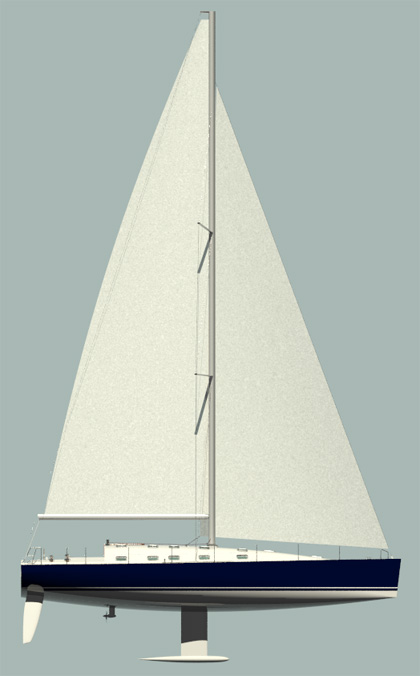VK45 Technical data Length overall = 13,6m Length waterline = 13,1m Beam overall = 4,15m Beam waterline = 3,1m Depth midsection = 3,7m Free board midsection = 1,4m Draft = 2,32m Displacement = 7950kg Ballast = 2350kg Engine M4.55-38.3kW(52hp) Mainsail = 61m2 Genoa = 57m2 Spinnaker = 170m2 I = 16.5m J = 6.1m P = 17.6m E = 5.9m Windward balance point at RM30:    RM30 = 53,6kNm    Yaw = 5.4degrees     V_wind = 18.3knots     V_hull = 8.4knots Water = 264l Calorifier = 45l Fuel = 122l Gray&black = 120l Boat design category CE: A+ Certification: Ocean plus Design: VK Yacht

Stability curve